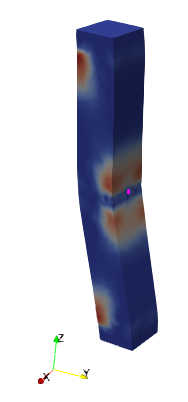# Stress direction in saved data (.csv)

Hi everyone…
I’ve extract or SaveData to csv. In the csv file, there are 9 stress types (Stress0 to Stress8 and StressMagnitude). Could anyone please help me, where can I find the explanation of these stresses especially direction of each stress type. I found the pairs between these stresses (1-3, 2-6, and 5-7), which has similar value within the columns of each pair.
Please let me know the explanation of it.
Thanks

These stresses comes from somwhere, either a filter or your initial dataset. You need to share more info.

Hi @sandyITS,

It looks like you are exporting a stress tensor (Cauchy stress tensor - Wikipedia) from your data. Is this data from a mechanical simulation?

In this case it is most likely that each row of your csv file represents one component of the stress tensor (3 x 3 = 9). And the similarities between different components is because the tensor is symmetric.

The magnitude of the stress tensor is often defined as the square root of the dot product of the tensor with itself similarly to matrices (Matrix norm - Wikipedia).

What exactly were you expecting from your stress data?

Best regards,

1 Like

Hi @mwestphal

CUBE.pvd (5.0 KB)
CUBE.pvsm (828.8 KB)
Paraview_SaveData.csv (129.5 KB)

Hi @jfausty

Yes, this is from mechanical simulation (.vtk files) about 50 steps, so I have 50 .vtk files.
Do you mean
Stress0 is SigmaX
Stress1 is ThetaXy
Stress2 is ThetaXz
Stress3 is SigmaY
Stress4 is ThetaYx
Stress5 is ThetaYz
Stress6 is SigmaZ
Stress7 is ThetaZx
Stress8 is ThetaZy
in that order?
I follow the above order and the pairs do not match at pair 5-7 because they are in different directions. Confuses me alot… I provide you the .csv file and the model.Paraview_SaveData.csv (129.5 KB)

Thankyou

Hi @sandyITS,

This actually truly depends on where your data comes from and how the source encodes tensor data. If that is the order in which your simulation wrote the components of the tensor than that is how they should be read in ParaView.

Usually, in my experience however, tensor components are ordered as so:

\sigma = \begin{pmatrix}\sigma_0 & \sigma_1 & \sigma_2 \\ \sigma_3 & \sigma_4 & \sigma_5 \\ \sigma_6 & \sigma_7 & \sigma_8 \end{pmatrix} = \begin{pmatrix}\sigma_x & \tau_{xy} & \tau_{xz} \\ & \sigma_y & \tau_{yz} \\ & & \sigma_z \end{pmatrix}

(taking into account symmetry)

This ordering would also support your previous observation that
\begin{array}{c c c}\sigma_{1} \simeq \sigma_{3} & \sigma_{2} \simeq \sigma_{6} & \sigma_{5} \simeq \sigma_{7} \end{array}

Best,